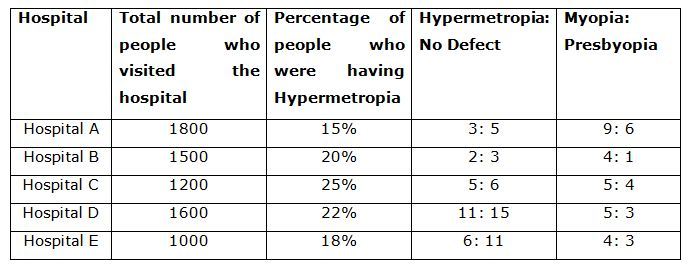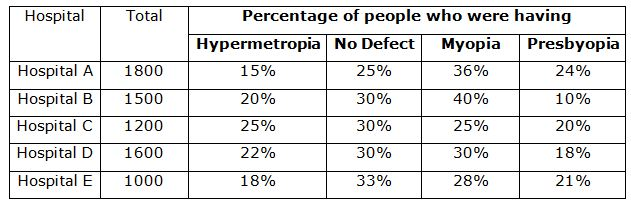# IBPS Clerk Mains Quantitative Aptitude Questions 2019 – (Day-1)

Dear Aspirants, Our IBPS Guide team is providing new pattern Quantitative Aptitude Questions for IBPS Clerk Mains 2019 so the aspirants can practice it on a daily basis. These questions are framed by our skilled experts after understanding your needs thoroughly. Aspirants can practice these new series questions daily to familiarize with the exact exam pattern and make your preparation effective.

Check here for IBPS Clerk Prelims Mock Test 2019

[WpProQuiz 7249]

Data Interpretation

Directions (1 – 5): Study the following information carefully and answer the given questions.

In a city, people with 3 types of eye defects (Myopia, Hypermetropia, and Presbyopia) visited different hospitals (Hospital A, Hospital B, Hospital C, Hospital D, and Hospital E). Some people who were having no eye defect also visited the hospitals. The total number of people visiting the hospital is given in the table. The percentage of people who were having Hypermetropia is given in the table along with the ratio of the number of people having Hypermetropia defects to the number of people having no defect. The ratio of the number of people who were having Myopia to the number of people who were having Pyesbyopia is also given in the table. Read the table carefully and answer the following questions.1) Find the total number of people who visited the hospital A and have Myopia.

a) 568

b) 648

c) 658

d) 638

e) 548

2) Find the ratio of the number of people who visited Hospital C and are having Presbyopia to the number of people who visited Hospital D and are having no defect.

a) 1: 2

b) 1: 3

c) 2: 3

d) 3: 4

e) 4: 5

3) Find the total number of people who visited all five Hospitals and have no eye defect.

a) 1070

b) 2050

c) 1970

d) 2070

e) 2270

4) Find the difference between the number of people who visited hospital E and have Myopia than the number of people who visited hospital E and have Presbyopia.

a) 50

b) 60

c) 70

d) 80

e) 90

5) Find the approximate overall percentage of people who visited the given hospital and have a defect of Hypermetropia. (Consider all five hospitals)

a) 12%

b) 16%

c) 18%

d) 20%

e) 22%

Application sums

6) Sathish, Simon and Raja completed a work together in 24 days and received a total payment of Rs. 30000. Sathish took half of the total payment, Simon took one third and Raja took the remaining amount. In how many days, Sathish and Raja would have finished the work if Simon was not working?

a) 12 days

b) 24 days

c) 36 days

d) 48 days

e) None of these

7) The marked price of a doll is 45% above its manufacturing cost. The doll is sold through a retailer, who earns 10% profit on his purchase price. The retailer gives 20% discount on marked price. What is the approximate profit percentage for the doll manufacturer who sells his doll to the retailer?

a) 5%

b) 8%

c) 10%

d) 15%

e) 12%

8) The distance between two schools of Madurai and Trichy is 150 km. A car starts from Madurai and moves towards Trichy at an average speed of 10 km/h. Another car starts from Trichy, 10 minutes earlier than the first car and moves towards Madurai at an average speed of 15 km/h. How far from Madurai and from Trichy will the two cars will meet respectively?

a) 59km and 91km

b) 65km and 85km

c) 40km and 110km

d) 55km and 95km

e) None of these

9) Two trains each having a length of 200 meters moving in opposite direction crossed each other in 10 seconds. If first train crossed a 250 metre long platform in 30 seconds, then the ratio of their speeds is:

a) 1: 3

b) 3: 5

c) 2: 3

d) 4: 3

e) None of these

10) Three sugar types of Rs. 45 per kg, Rs. 75 per kg and Rs. 50 per kg are mixed in a particular ratio. Find the ratio if the resultant mixture is sold at 77% of highest sugar price type to make 10% profit.

a) 5: 3: 2

b) 3: 2: 8

c) 5: 1: 4

d) 3: 2: 9

e) 1: 1: 4

Directions (1-5):

Hospital A:

Total number of people who visited Hospital A = 1800

Percentage of people having Hypermetropia defect (Hospital A) = 15%

Percentage of people with no defect (Hospital A) = 15% * (5/3) = 25%

Percentage of people having Myopia or Presbyopia (Hospital A)

= 100% – (15% + 25%) = 100% – 40% = 60%

Percentage of people having Myopia defect (Hospital A) = 60% * {9/(9 + 6)}

= 60% * (9/15) = 36%

Percentage of people having Presbyopia defect (Hospital A) = 60% * {6/(9 + 6)}

= 60% * 6/15 = 24%

Similarly calculate the percentage of all the hospitals.Note: Total number of people who visited the hospital = People having Hypermetropia + People having No defect + People having Myopia + People having Presbyopia

Number of people having Myopia (Hospital A) = 36% of 1800 = 648

Number of people having Presbyopia (Hospital C) = 20% of 1200 = 240

Number of people having no defect (Hospital D) = 30% of 1600 = 480

Required ratio = 240: 480 = 1: 2

Number of people how visited Hospital A and have no eye defect

= 25% of 1800 = 450

Number of people how visited Hospital B and have no eye defect

= 30% of 1500 = 450

Number of people how visited Hospital C and have no eye defect

= 30% of 1200 = 360

Number of people how visited Hospital D and have no eye defect

= 30% of 1600 = 480

Number of people how visited Hospital E and have no eye defect

= 33% of 1000 = 330

Therefore, the total number of people who visited all five Hospitals and have no eye defect

= (450 + 450 + 360 + 480 + 330)

= 2070

Required difference = 1000 * (28% – 21%)

= 1000 * 7/100 = 70

Number of people how visited Hospital A and have Hypermetropia = 15% of 1800

= 270

Number of people how visited Hospital B and have Hypermetropia= 20% of 1500

= 300

Number of people how visited Hospital C and have Hypermetropia= 25% of 1200

= 300

Number of people how visited Hospital D and have Hypermetropia= 22% of 1600

= 352

Number of people how visited Hospital E and have Hypermetropia = 18% of 1000

= 180

Therefore, the total number of people who visited all five Hospitals and have Hypermetropia

= 270 + 300 + 300 + 352 + 180

= 1402

Total number of people who visited all five hospitals

= 1800 + 1500 + 1200 + 1600 + 1000 = 7100

Required percentage = (1402/7100) * 100 = 20% (Approx.)

Directions (6-10):

Sathish, Simon and Raja completed a work together in 24 days

Ratio of the shares of Sathish, Simon and Raja= 1/2: 1/3: (1 – ½ – 1/3)

= 3: 2: 1

Work done by Sathish, Simon and Raja in one day is 3T, 2T and T, respectively.

=> 3T + 2T + T = 1/24

=> T = 1/144

Work done by Sathish in one day = 3T = 1/48

Work done by Raja in one day = T = 1/144

Let us suppose that when Sathish and Raja work together, they take n days to finish the work.

N/48 + N/144 = 1

=> N = 36 days

When Sathish and Raja work together, they take 36 days to finish the work.

Let the manufacturing cost = 100 and manufacturer profit = x

Marked price of the doll= 145% of 100 = 145

The retailer gives 20% discount on marked price, Buyer Price = 80% of 145 = 116

Manufacturer makes x rupees profit, and then retailer makes 10% profit.

Hence, 110% of (100 + x) = 116

⇒ 110 (100 + x) = 11600

⇒ 11000 + 110x = 11600

⇒ x = 5.45%. Hence, Manufacturer profit = 5.45% ≈ 5%

Let t = time at which the two cars met each other.

If the car starting from Madurai have travelled for time “t” hr, then the car starting from Trichy will have travelled for [t + (10/60)]hr.

Hence we get, (10 * t) + 15 * [t + (10/60)] = 150

=> 10 * t + 15 * t + 15 * 10/60 = 150

=> t = 59/10 hr.

Distance from Madurai when the two cars will met= 10 * 59/10 = 59km

Distance from Trichy when the two cars met = 150km – 59km = 91km

Let the speed of first train be ‘a’ m/sec and that of second train be ‘b’ m/sec.

Then, we get the below two equations,

10 = (200 + 200)/ (a + b)

=> a + b = 400/10

=> a + b = 40 —- (i)

30 = (200 + 250)/a

=> a = 15 —- (ii)

By substituting a = 15 in (i), we get b = 25

a = 15m/s, b = 25m/s

Required ratio = a: b = 15: 25 = 3: 5

Selling price = 77% of highest sugar price = (77/100) * 75 = 57.75

Profit percent = 10%

SP/CP = 110/100; CP = 100 * 57.75/110 = Rs.52.5

Take first two quantities 45 and 75

Ratio of these two = (75 – 52.5)/(52.5 – 45) = 22.5/7.5 = 3/1 = 3: 1

Take second and third quantities,

Ratio of these two = (52.5 – 50)/(75 – 52.5) = 2.5/22.5 = 1/9 = 1: 9

These two mixtures cost 52.5 per kg. So when these two mixtures are mixed together in any ratio, we will get 52.5 rupees of mixture.

We will consider 1: 1 ratio of mixing Ratio = 3: 2: 9